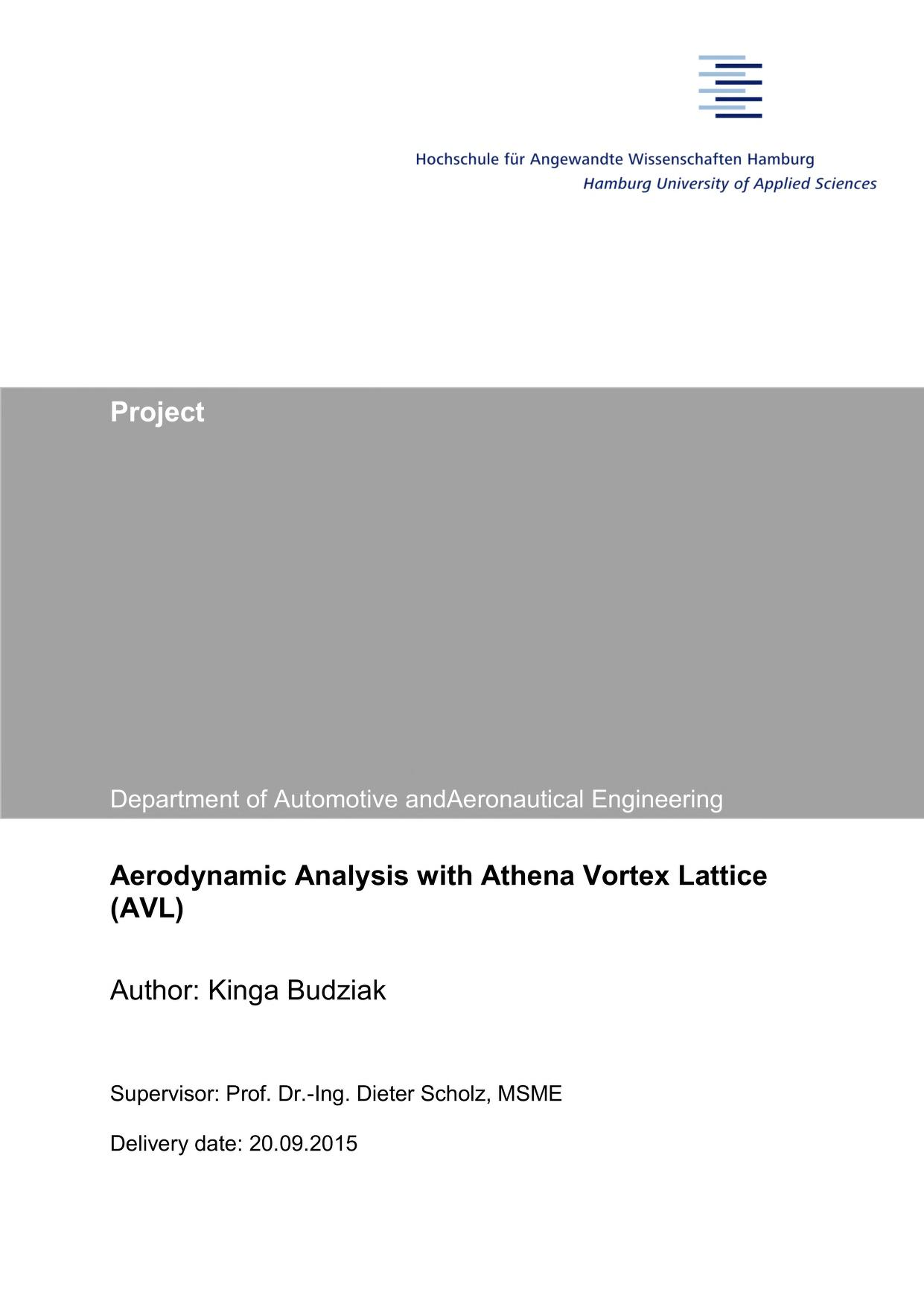Preview this item
Checking...

# Aerodynamic Analysis with Athena Vortex Lattice (AVL)

Author: Kinga Budziak; Dieter Scholz Aircraft Design and Systems Group (AERO), Department of Automotive and Aeronautical Engineering, Hamburg University of Applied Sciences, Hamburg, Germany, 2015 Digital Library - Projects & Theses - Prof. Dr. Scholz; http://library.ProfScholz.deDownloadable archival material : English This project evaluates the suitability and practicality of the program Athena Vortex Lattice (AVL) by Mark Drela. A short user guide was written to make it easier (especially for students) to get started with the program AVL. AVL was applied to calculate the induced drag and the Oswald factor. In a first task, AVL was used to calculate simple wings of different aspect ratio A and taper ratio lambda. The Oswald factor was calculated as a function f(lambda) in the same way as shown by HOERNER. Compared to HOERNER's function, the error never exceed 7.5%. Surprisingly, the function f(lambda) was not independent of aspect ratio, as could be assumed from HOERNER. Variations of f(lambda) with aspect ratio were studied and general results found. In a second task, the box wing was investigated. Box wings of different h/b ratio: 0.31, 0.62, and 0.93 were calculated in AVL. The induced drag and Oswald factor in all these cases was calculated. An equation, generally used in the literature, describes the box wing's Oswald factor with parameters k1, k2, k3 and k4. These parameters were found from results obtained with AVL by means of the Excel Solver. In this way the curve k = f(h/b) was plotted. The curve was compared with curves with various theories and experiments conducted prior by other students. The curve built based on AVL fits very well with the curve from HOERNER, PRANDTL and a second experiment made in the wind tunnel at HAW Hamburg.  Read more... (not yet rated) 0 with reviews - Be the first.

## Find a copy in the libraryFinding libraries that hold this item...

## Details

Material Type: Internet resource Internet Resource, Archival Material Kinga Budziak; Dieter Scholz Find more information about: Kinga Budziak Dieter Scholz 1086581141 English Copyright by authorCC BY-NC-SAhttps://creativecommons.org/licenses/by-nc-sa/4.0 Digital Library - Projects & Theses - Prof. Dr. Scholz; http://library.ProfScholz.de

### Abstract:

This project evaluates the suitability and practicality of the program Athena Vortex Lattice (AVL) by Mark Drela. A short user guide was written to make it easier (especially for students) to get started with the program AVL. AVL was applied to calculate the induced drag and the Oswald factor. In a first task, AVL was used to calculate simple wings of different aspect ratio A and taper ratio lambda. The Oswald factor was calculated as a function f(lambda) in the same way as shown by HOERNER. Compared to HOERNER's function, the error never exceed 7.5%. Surprisingly, the function f(lambda) was not independent of aspect ratio, as could be assumed from HOERNER. Variations of f(lambda) with aspect ratio were studied and general results found. In a second task, the box wing was investigated. Box wings of different h/b ratio: 0.31, 0.62, and 0.93 were calculated in AVL. The induced drag and Oswald factor in all these cases was calculated. An equation, generally used in the literature, describes the box wing's Oswald factor with parameters k1, k2, k3 and k4. These parameters were found from results obtained with AVL by means of the Excel Solver. In this way the curve k = f(h/b) was plotted. The curve was compared with curves with various theories and experiments conducted prior by other students. The curve built based on AVL fits very well with the curve from HOERNER, PRANDTL and a second experiment made in the wind tunnel at HAW Hamburg.

## Reviews

User-contributed reviewsBe the first.

## Similar Items

### Related Subjects:(17)

Confirm this request

You may have already requested this item. Please select Ok if you would like to proceed with this request anyway.